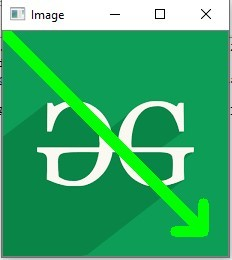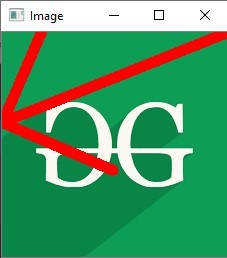# Python OpenCV | cv2.arrowedLine() method

• Last Updated : 23 Nov, 2021

OpenCV-Python is a library of Python bindings designed to solve computer vision problems.
cv2.arrowedLine() method is used to draw arrow segment pointing from the start point to the end point.

Syntax: cv2.arrowedLine(image, start_point, end_point, color, thickness, line_type, shift, tipLength)
Parameters:
image: It is the image on which line is to be drawn.
start_point: It is the starting coordinates of line. The coordinates are represented as tuples of two values i.e. (X coordinate value, Y coordinate value).
end_point: It is the ending coordinates of line. The coordinates are represented as tuples of two values i.e. (X coordinate value, Y coordinate value).
color: It is the color of line to be drawn. For BGR, we pass a tuple. eg: (255, 0, 0) for blue color.
thickness: It is the thickness of the line in px
line_type: It denotes the type of the line for drawing.
shift: It denotes number of fractional bits in the point coordinates.
tipLength: It denotes the length of the arrow tip in relation to the arrow length.
Return Value: It returns an image.

Image used for all the below examples:Example #1:

## Python3

 `# Python program to explain cv2.arrowedLine() method `   `# importing cv2 ` `import` `cv2 `   `# path ` `path ``=` `r``'C:\Users\Atomix\Desktop\geeksforgeeks\geeks.png'`   `# Reading an image in default mode ` `image ``=` `cv2.imread(path) `   `# Window name in which image is displayed ` `window_name ``=` `'Image'`   `# Start coordinate, here (0, 0) ` `# represents the top left corner of image ` `start_point ``=` `(``0``, ``0``) `   `# End coordinate` `end_point ``=` `(``200``, ``200``) `   `# Green color in BGR ` `color ``=` `(``0``, ``255``, ``0``) `   `# Line thickness of 9 px ` `thickness ``=` `9`   `# Using cv2.arrowedLine() method ` `# Draw a diagonal green arrow line` `# with thickness of 9 px ` `image ``=` `cv2.arrowedLine(image, start_point, end_point,` `                                     ``color, thickness) `   `# Displaying the image ` `cv2.imshow(window_name, image) `

Output:Example #2:

## Python3

 `# Python program to explain cv2.arrowedLine() method `   `# importing cv2 ` `import` `cv2 `   `# path ` `path ``=` `r``'C:\Users\Atomix\Desktop\geeksforgeeks\geeks.png'`   `# Reading an image in default mode ` `image ``=` `cv2.imread(path) `   `# Window name in which image is displayed ` `window_name ``=` `'Image'`   `# Start coordinate, here (225, 0) ` `# represents the top right corner of image ` `start_point ``=` `(``225``, ``0``) `   `# End coordinate` `end_point ``=` `(``0``, ``90``) `   `# Red color in BGR ` `color ``=` `(``0``, ``0``, ``255``) `   `# Line thickness of 9 px ` `thickness ``=` `9`   `# Using cv2.arrowedLine() method ` `# Draw a red arrow line` `# with thickness of 9 px and tipLength = 0.5` `image ``=` `cv2.arrowedLine(image, start_point, end_point, ` `                    ``color, thickness, tipLength ``=` `0.5``) `   `# Displaying the image ` `cv2.imshow(window_name, image) `

Output:My Personal Notes arrow_drop_up
Recommended Articles
Page :